### 一、题目描述

输入: 2



输入: 10



• 2 <= n <= 58

### 二、题目解析

• 模拟：模拟题目的运行。
• 规律：尝试总结出题目的一般规律和特点。
• 匹配：找到符合这些特点的数据结构与算法。
• 边界：考虑特殊情况。

#### 1、模拟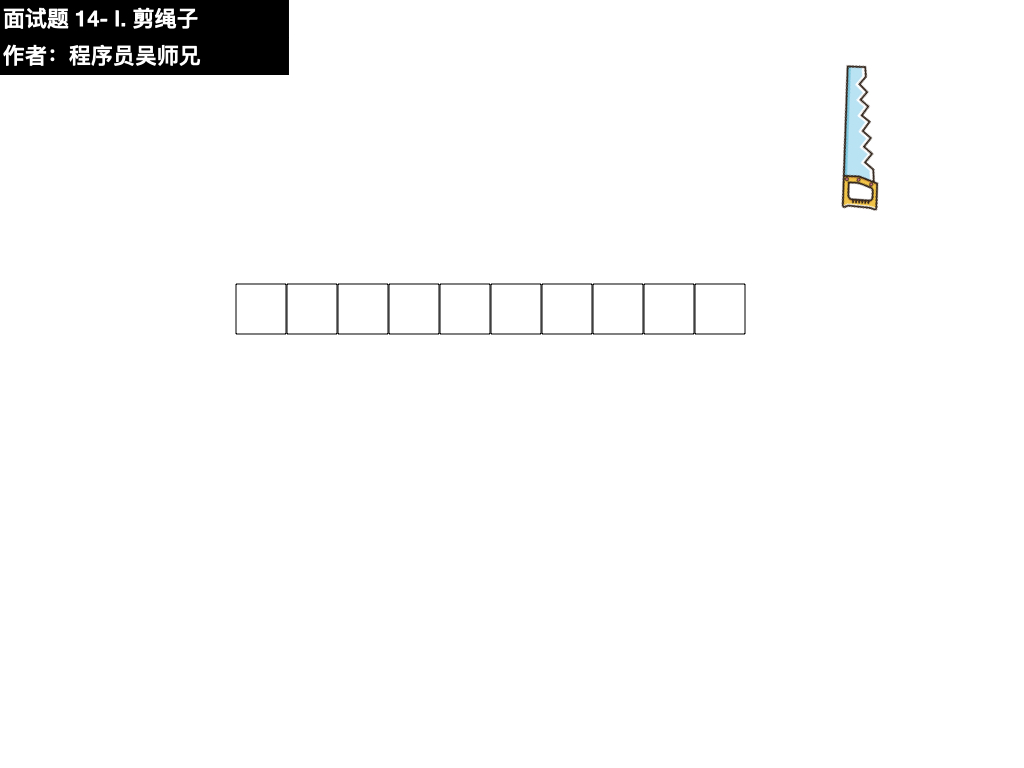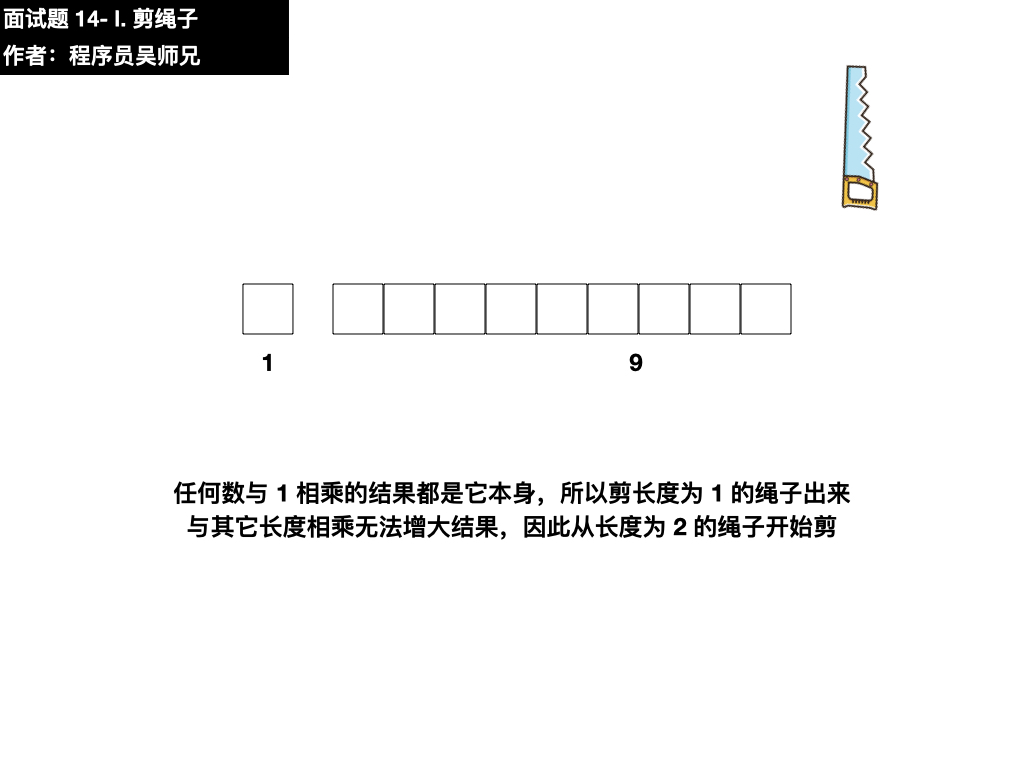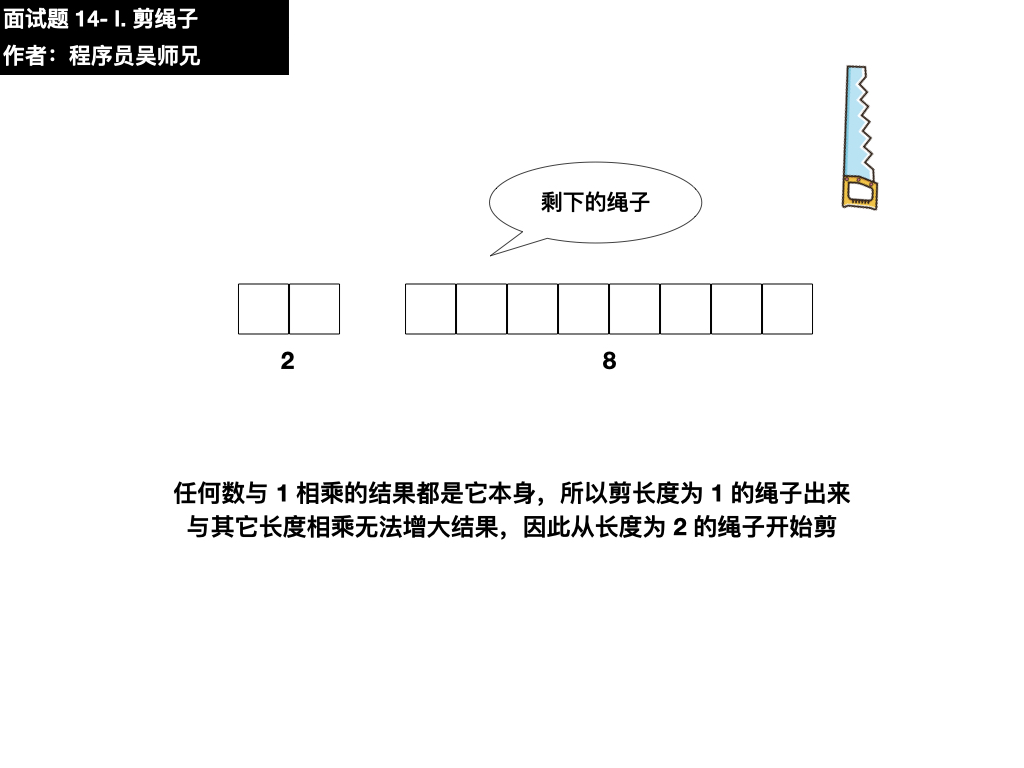• 不剪，乘积结果为 2 * 8 = 16
• 剪，怎么剪？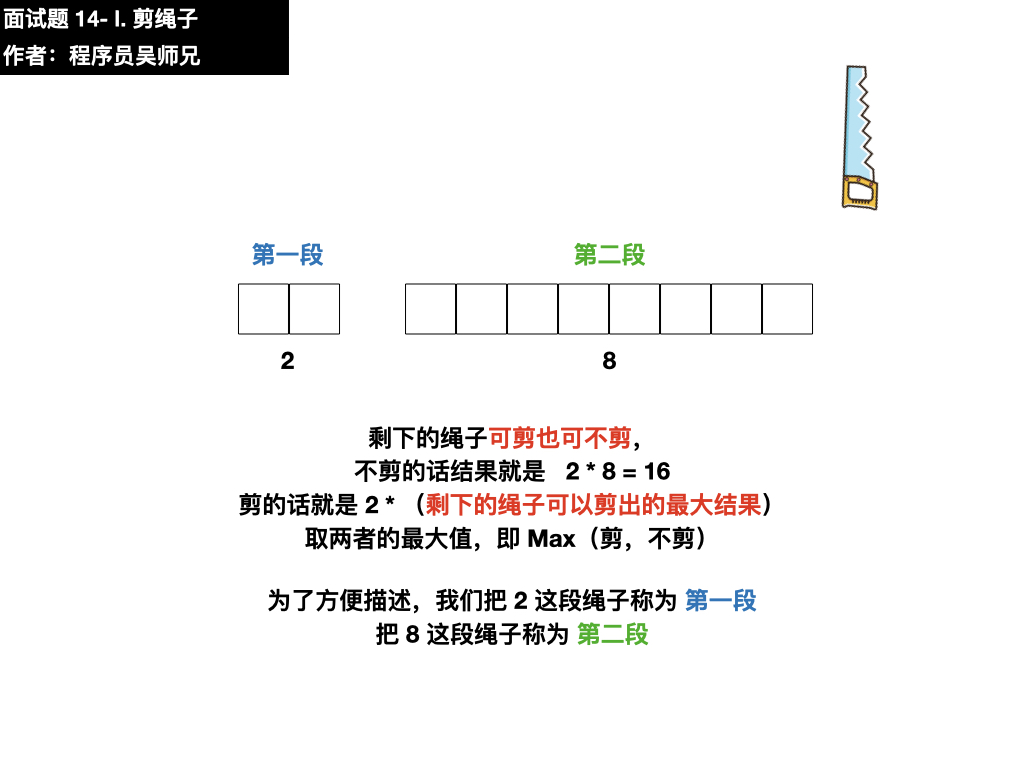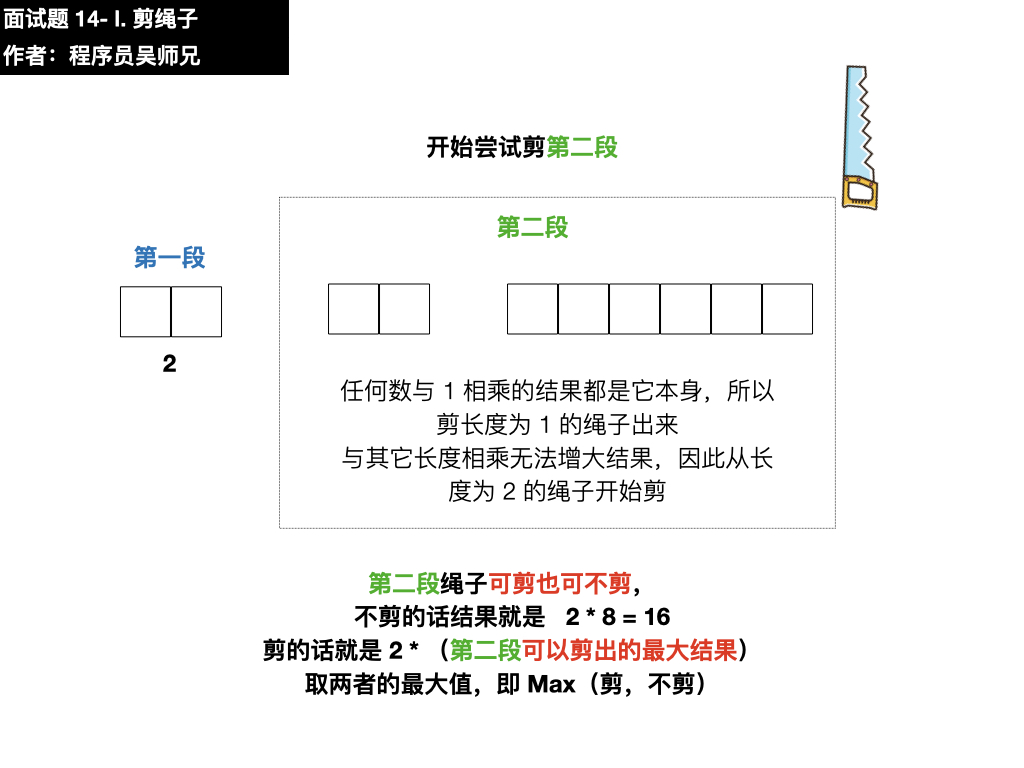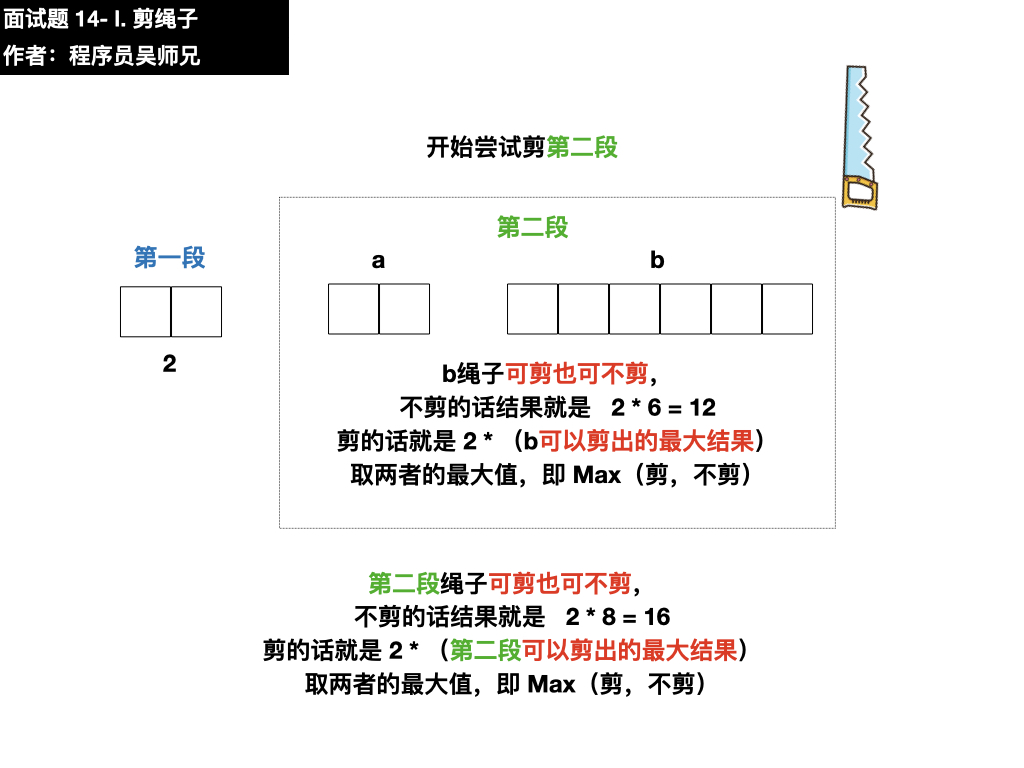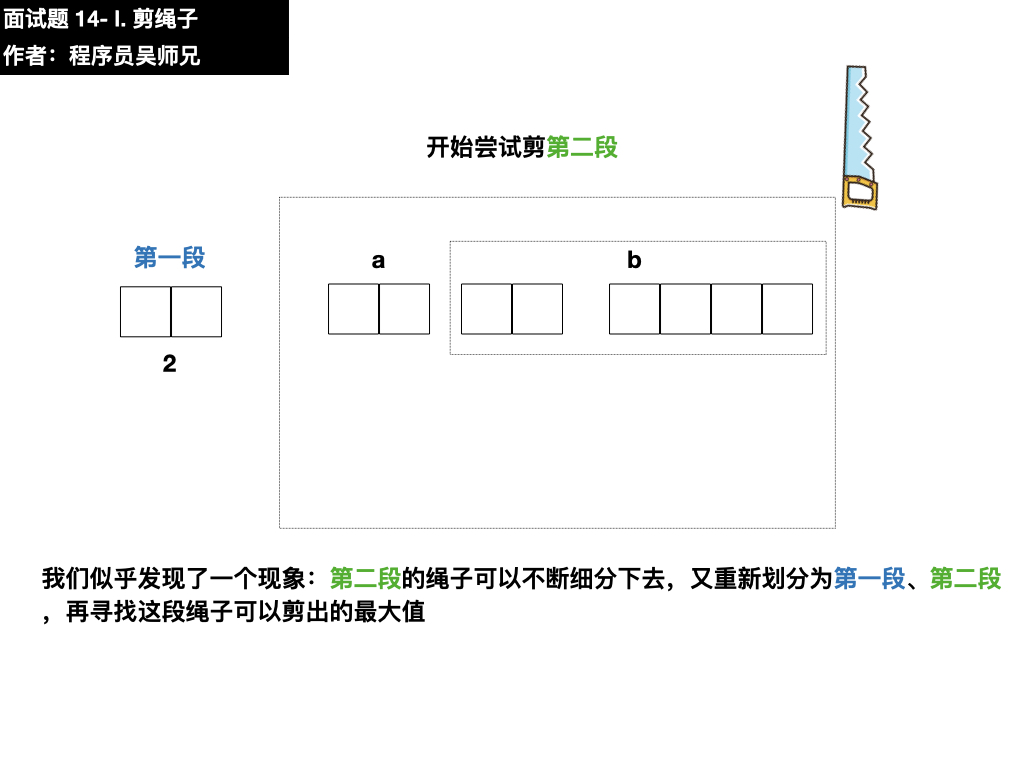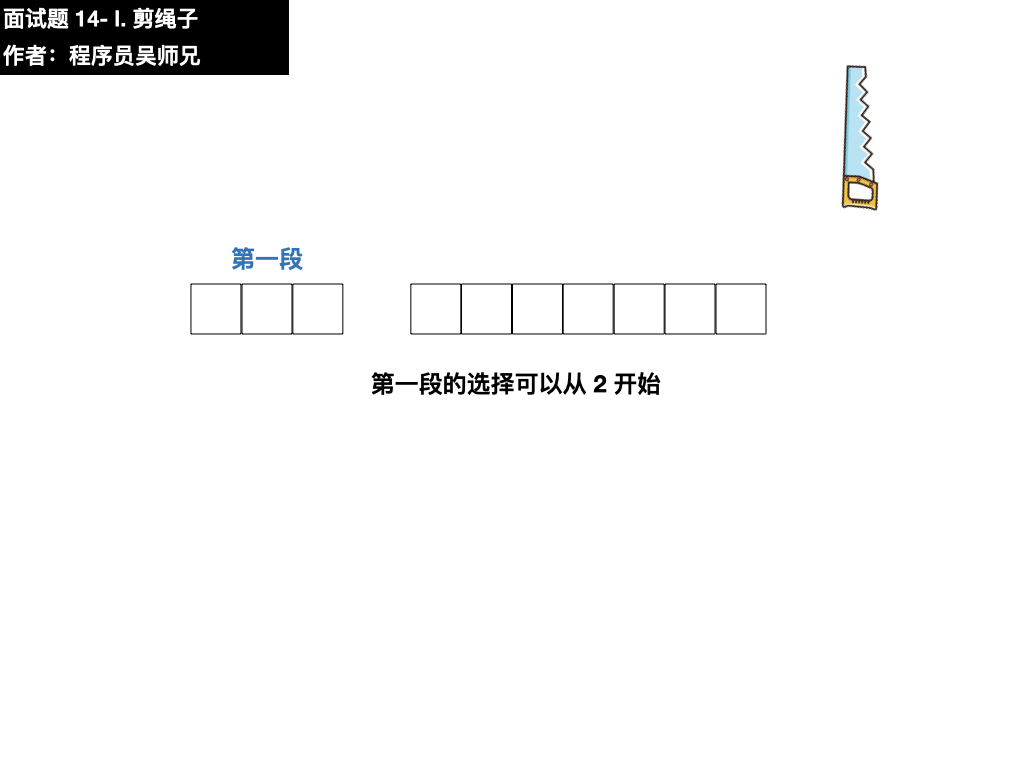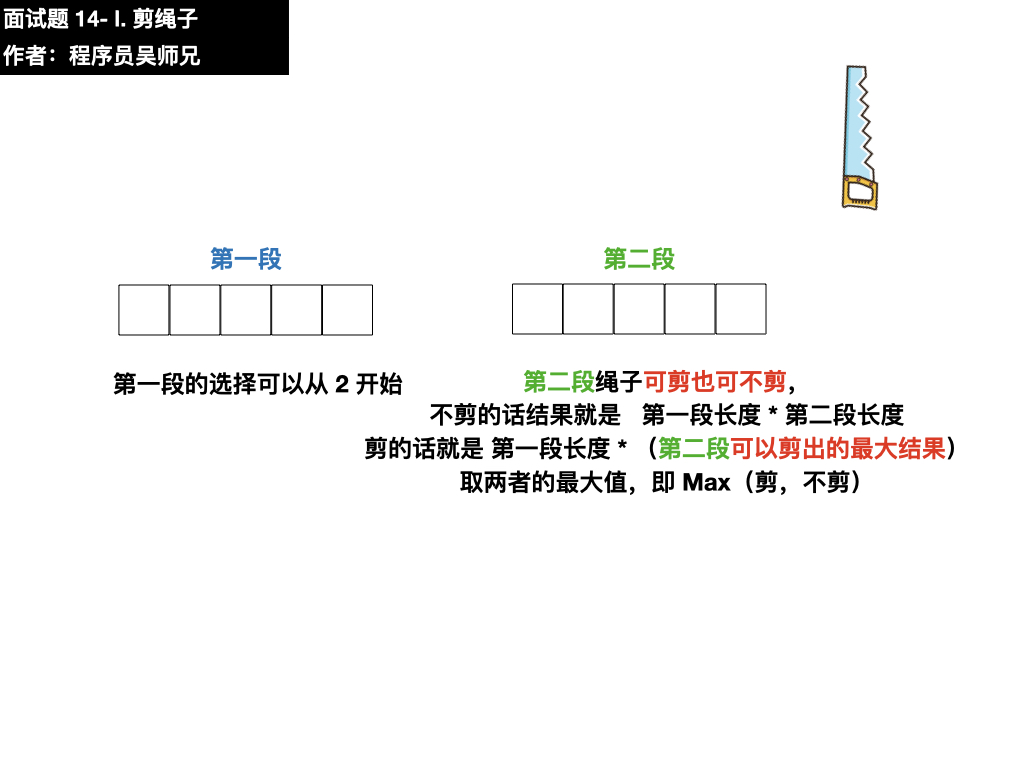#### 2、规律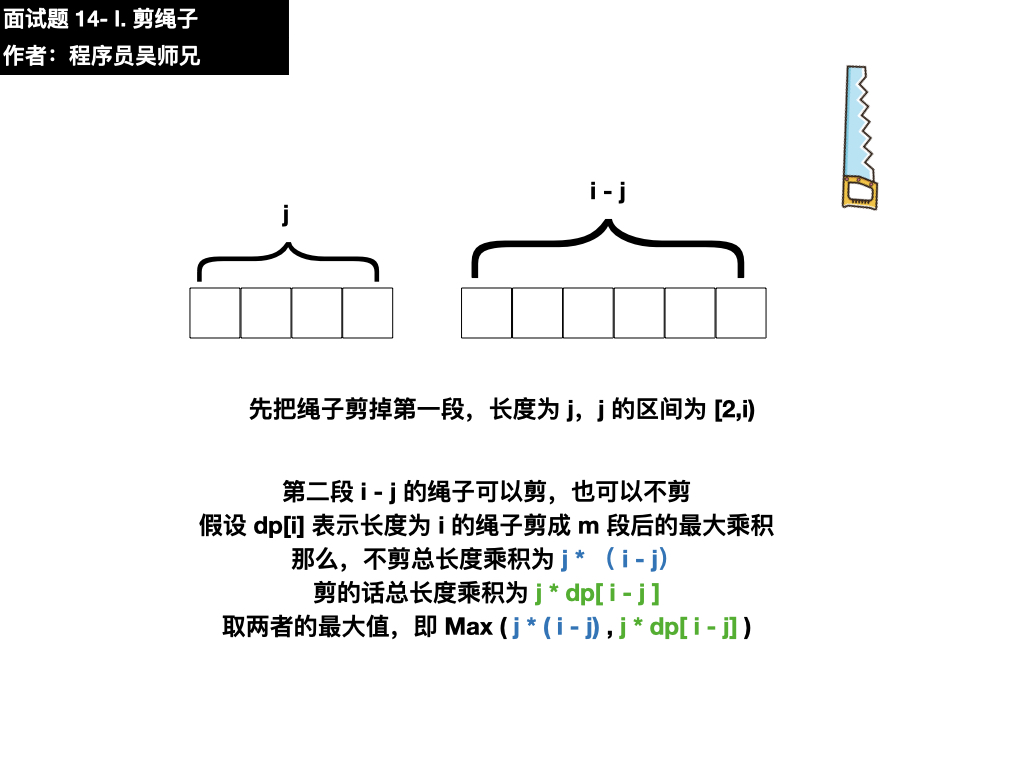#### 3、匹配

• （1）是求最优解问题，如最大值，最小值；
• （2）该问题能够分解成若干个子问题，并且子问题之间有重叠的更小子问题。

• 长度为 1 的绳子
• 长度为 2 的绳子

### 三、动画描述

• 普通用户特权：5积分
• 会员用户特权：免费
• 算法训练营永久会员用户特权：免费推荐

### 四、图片描述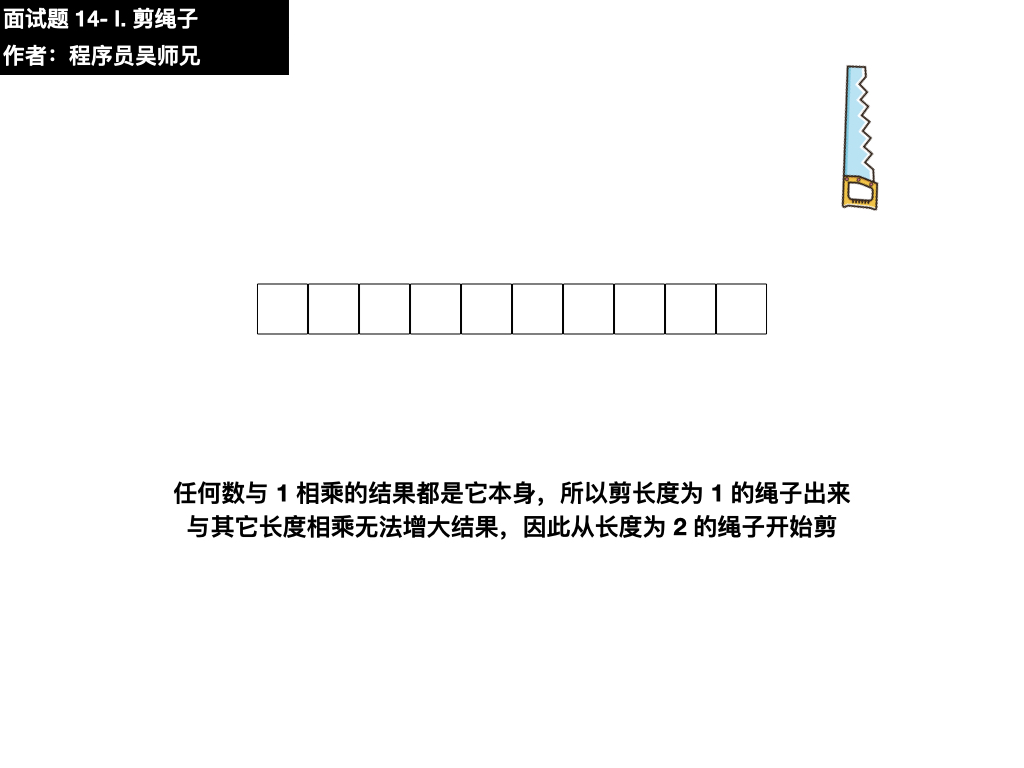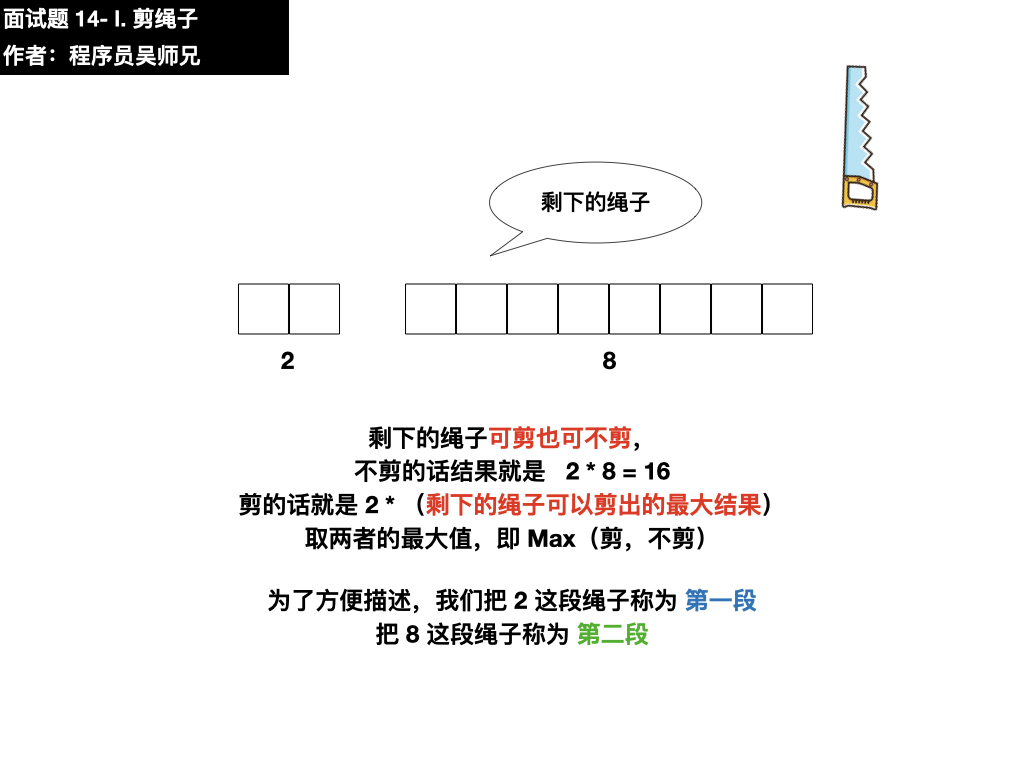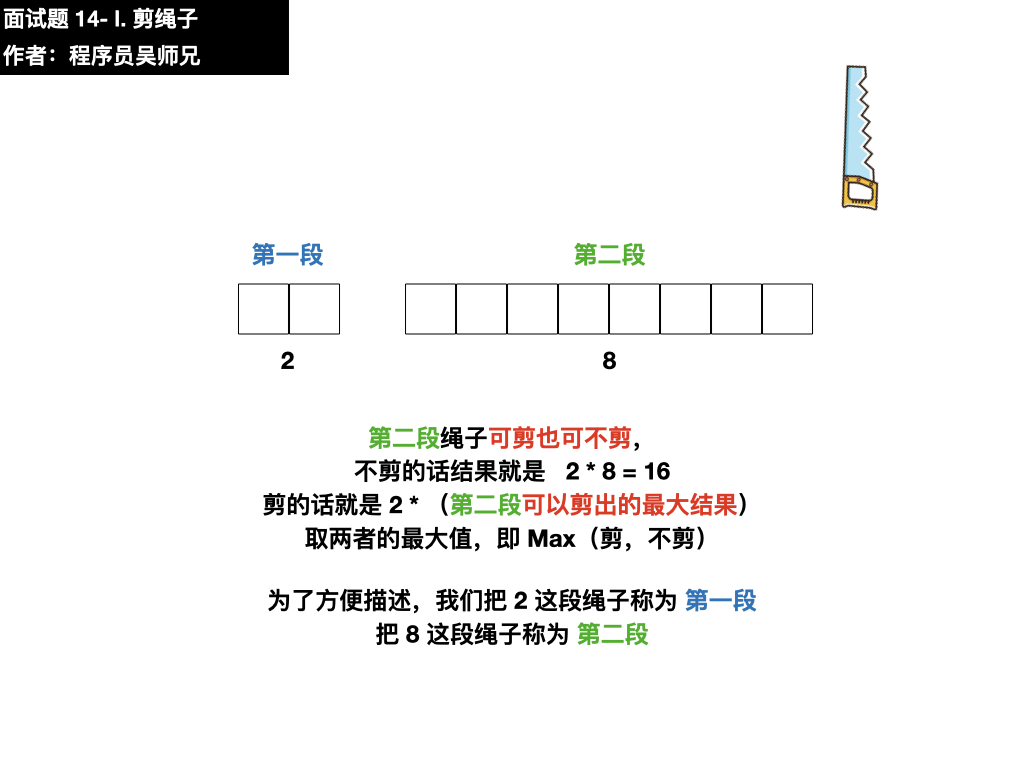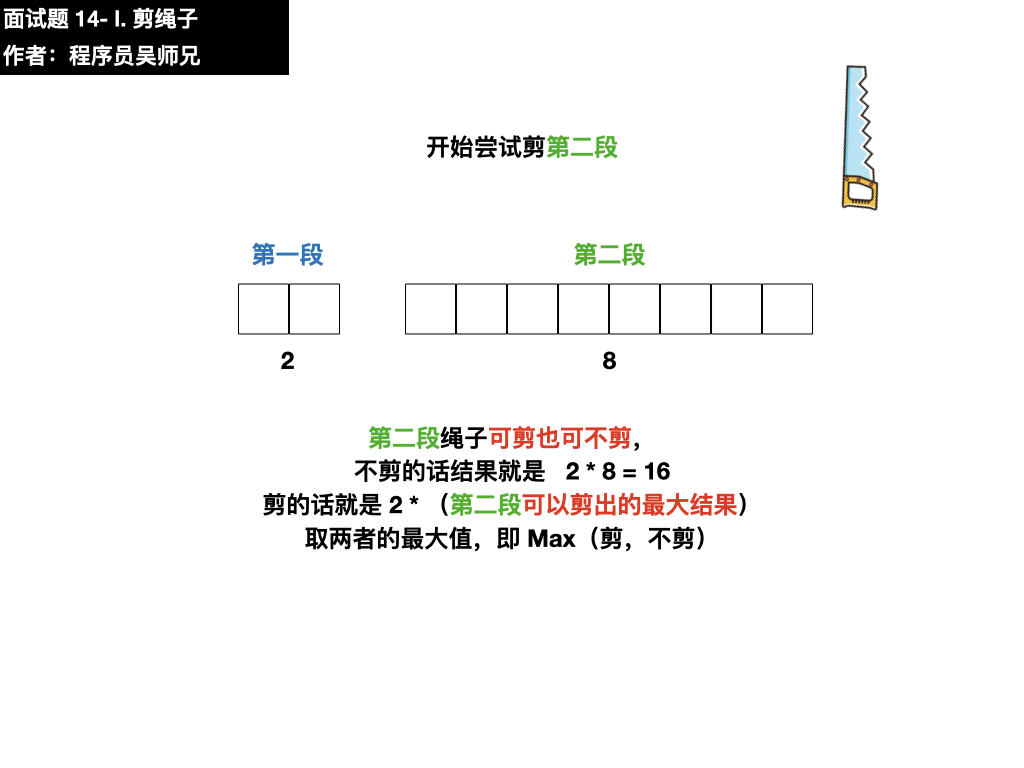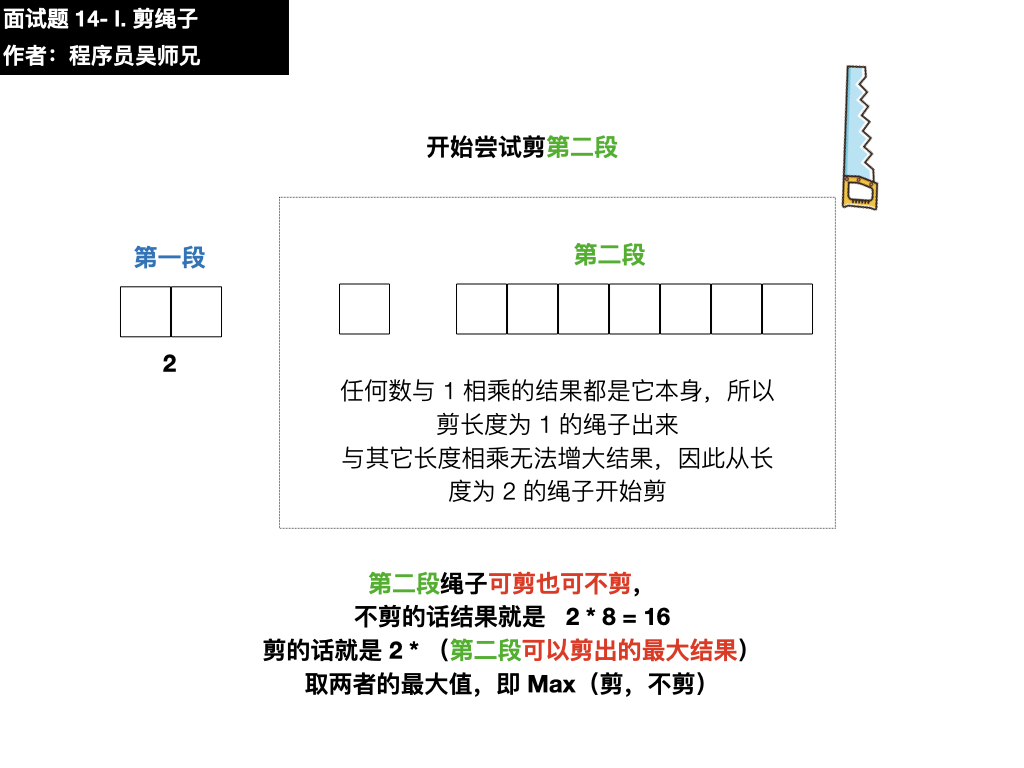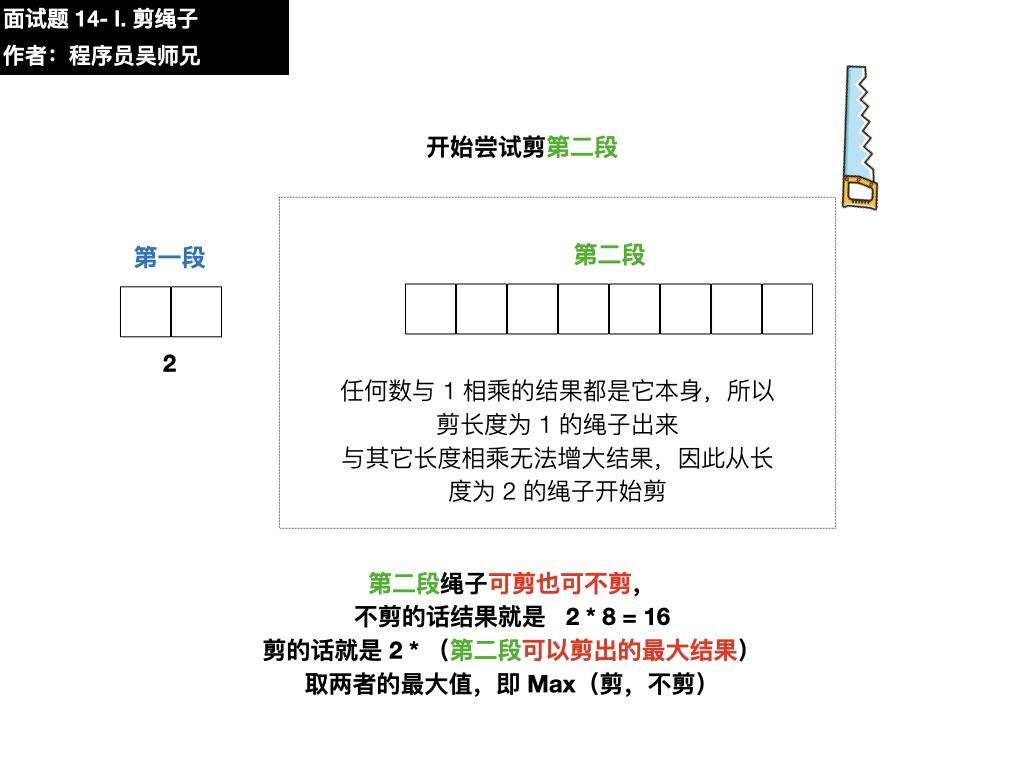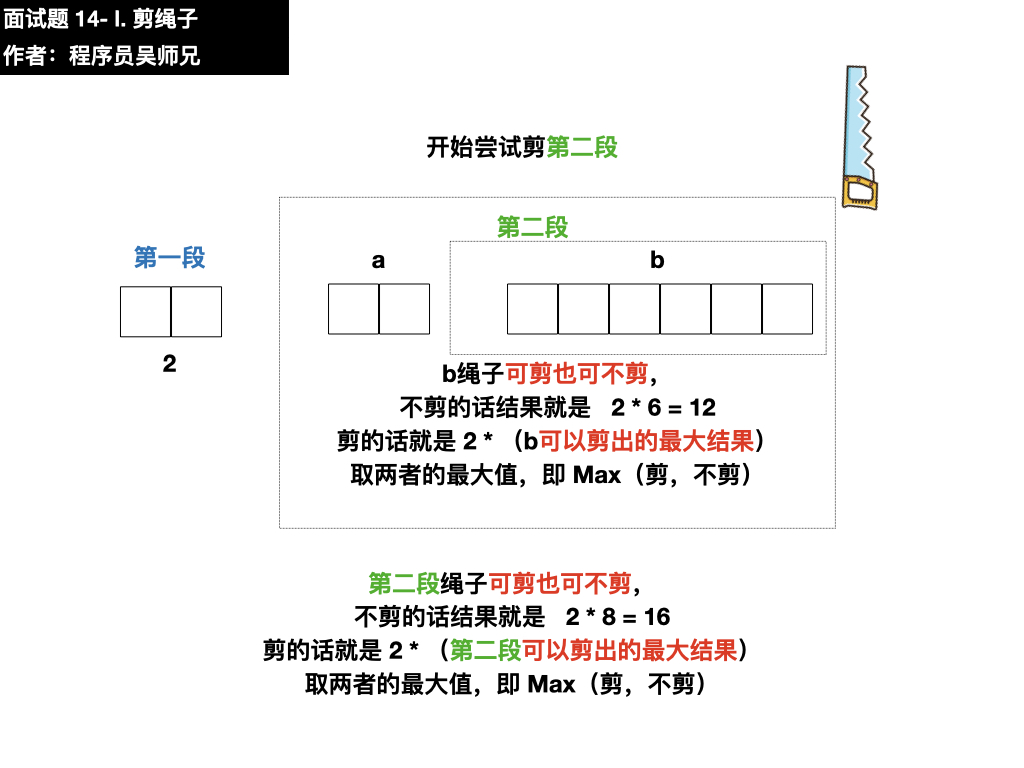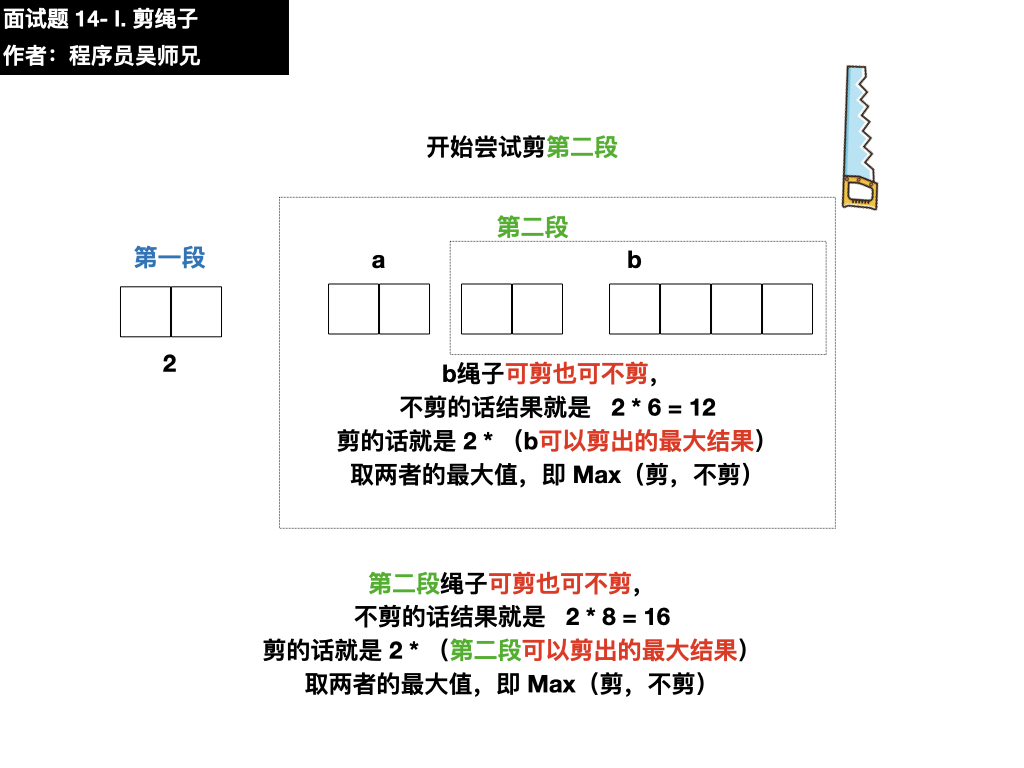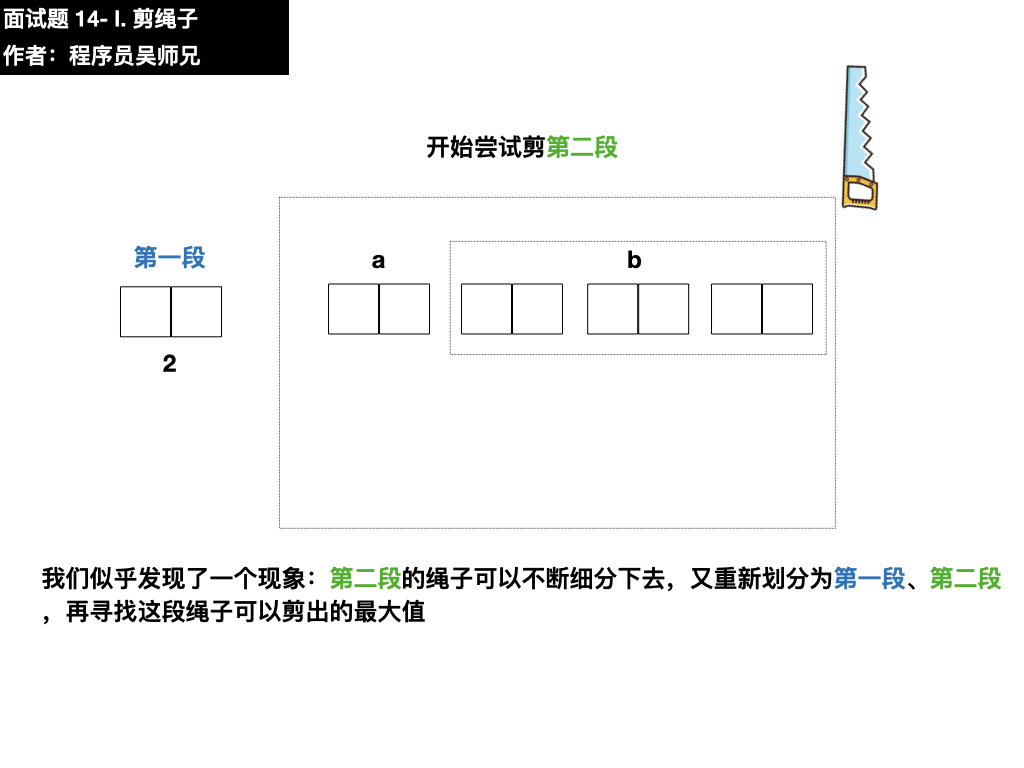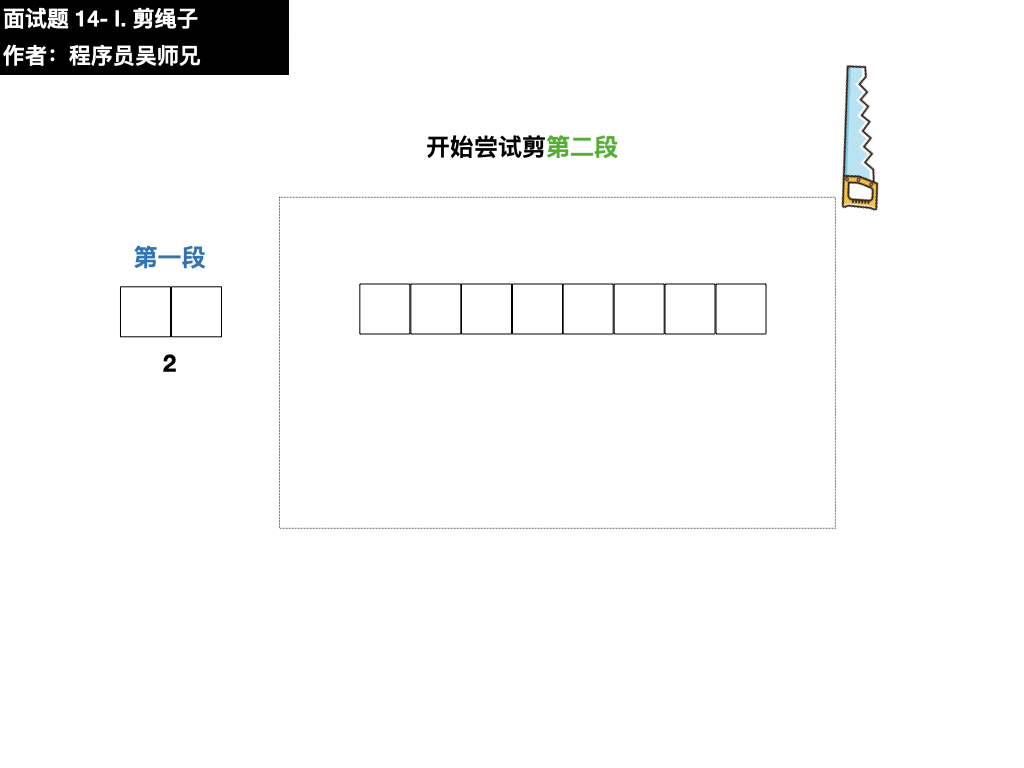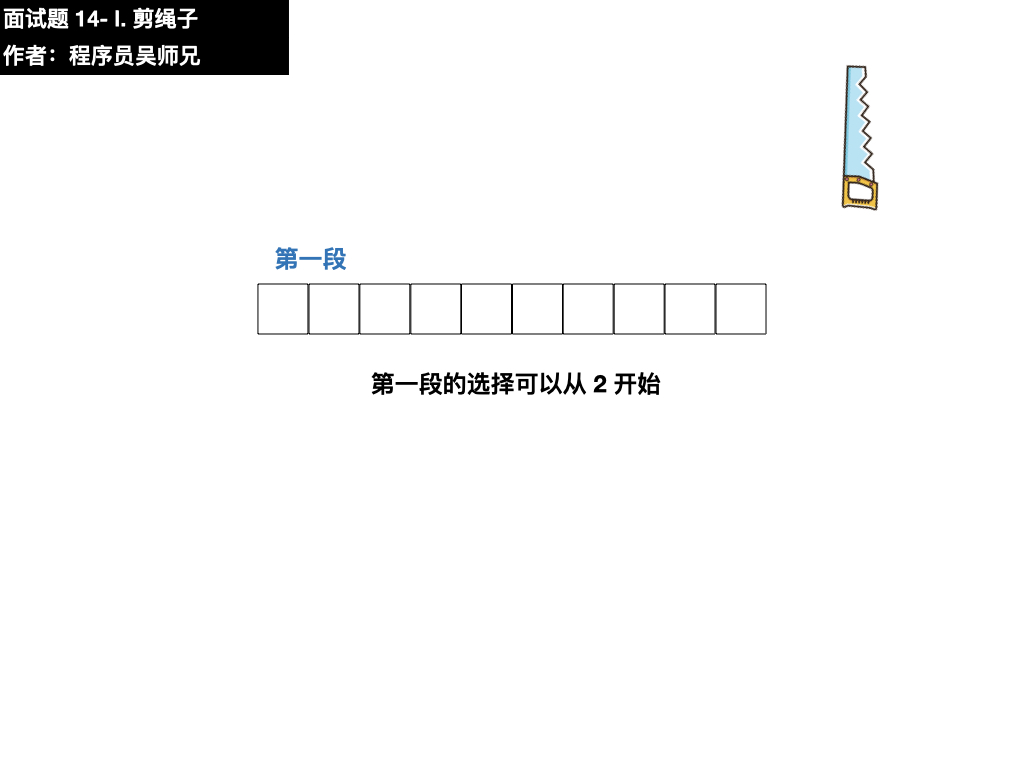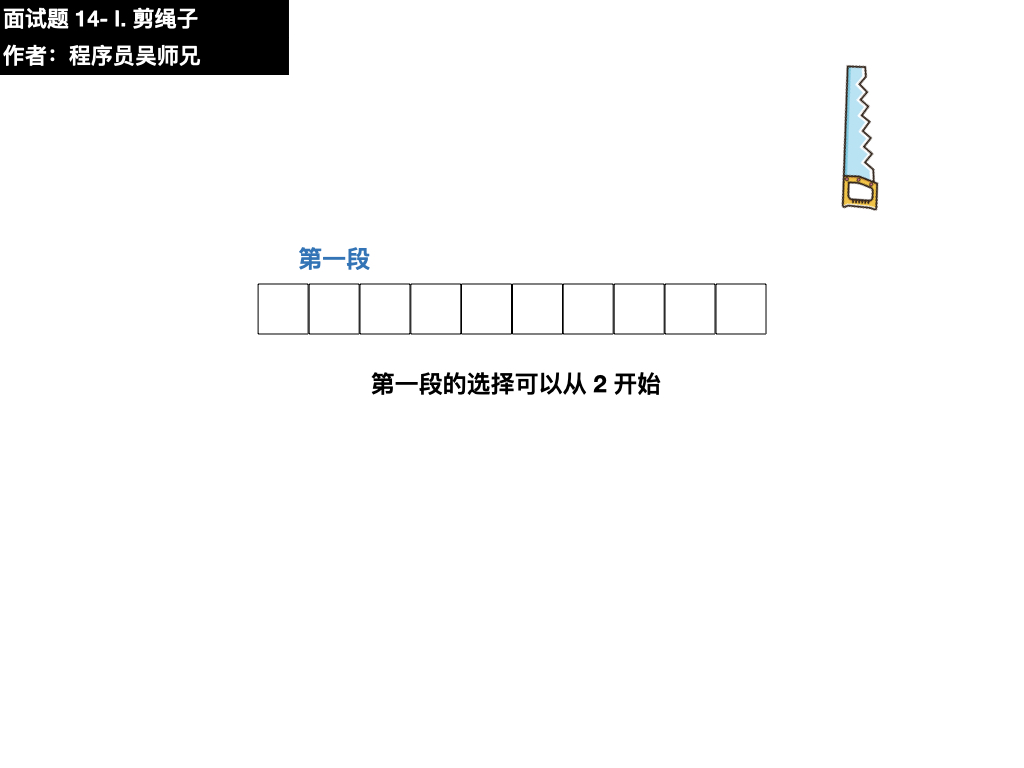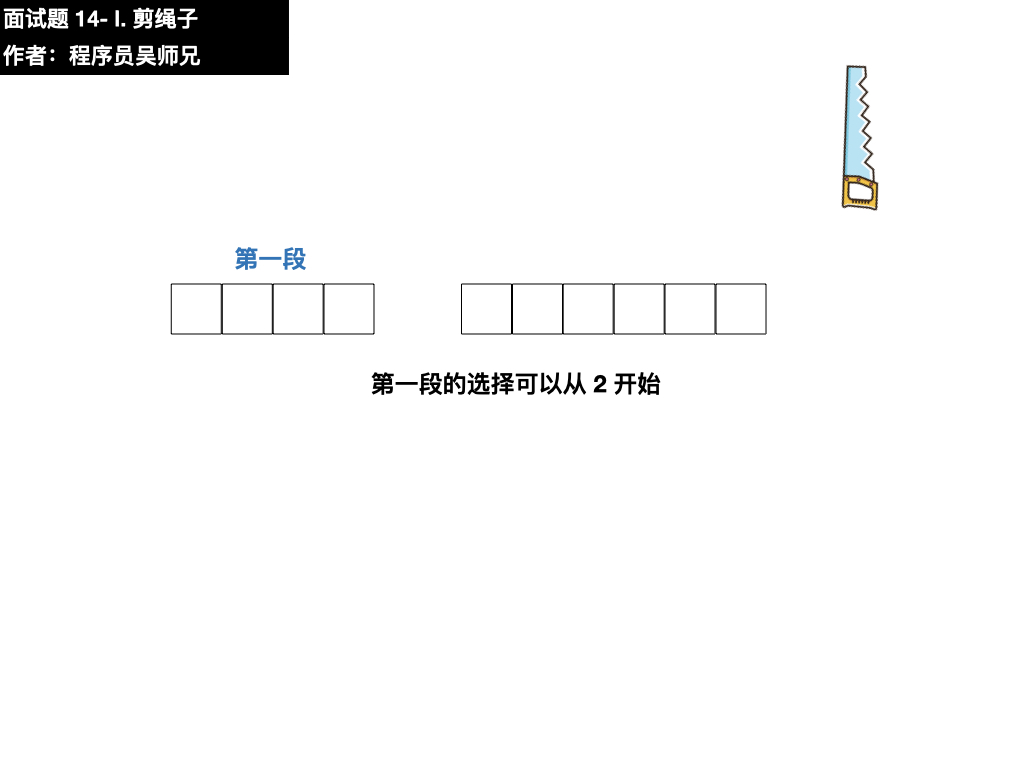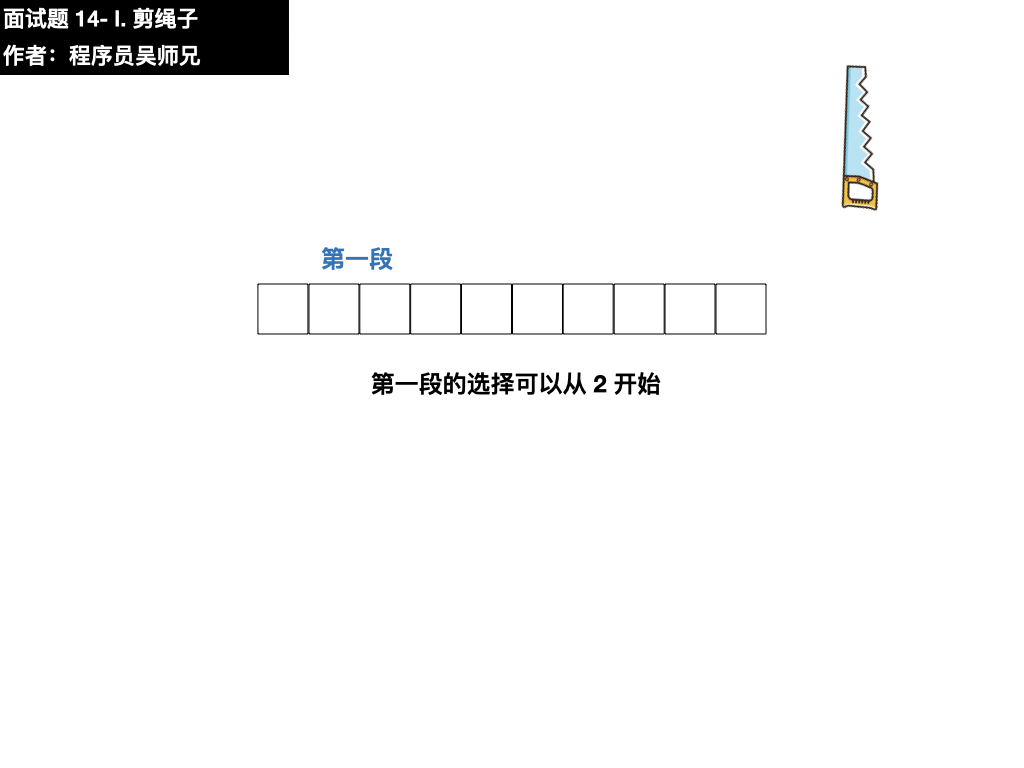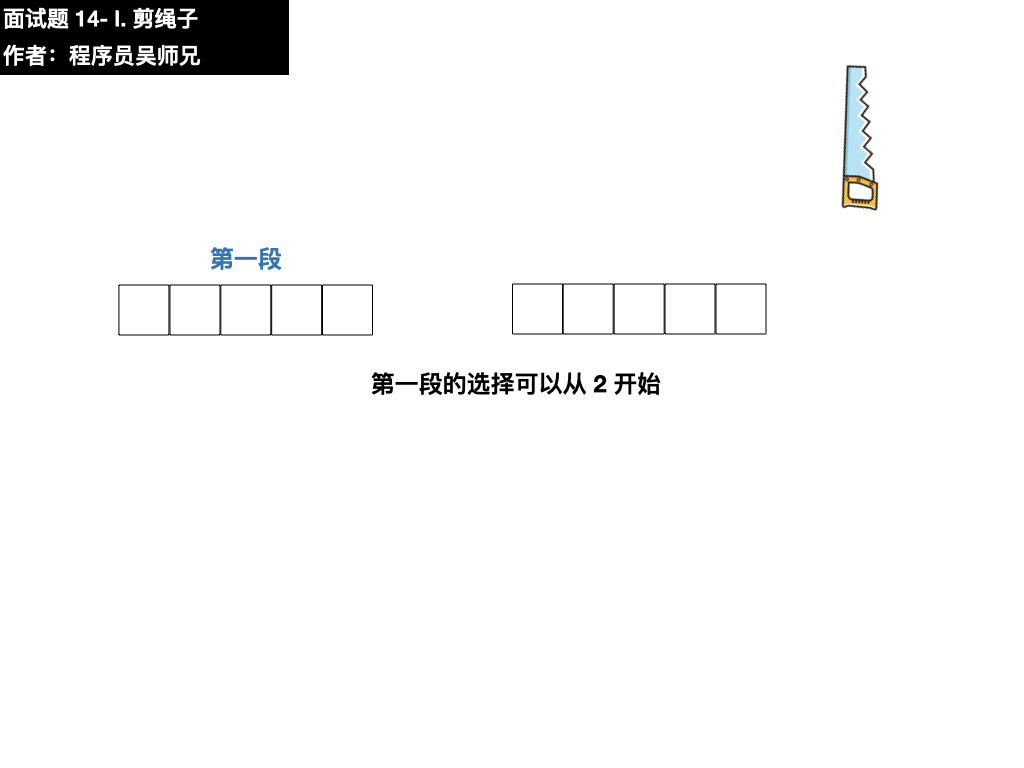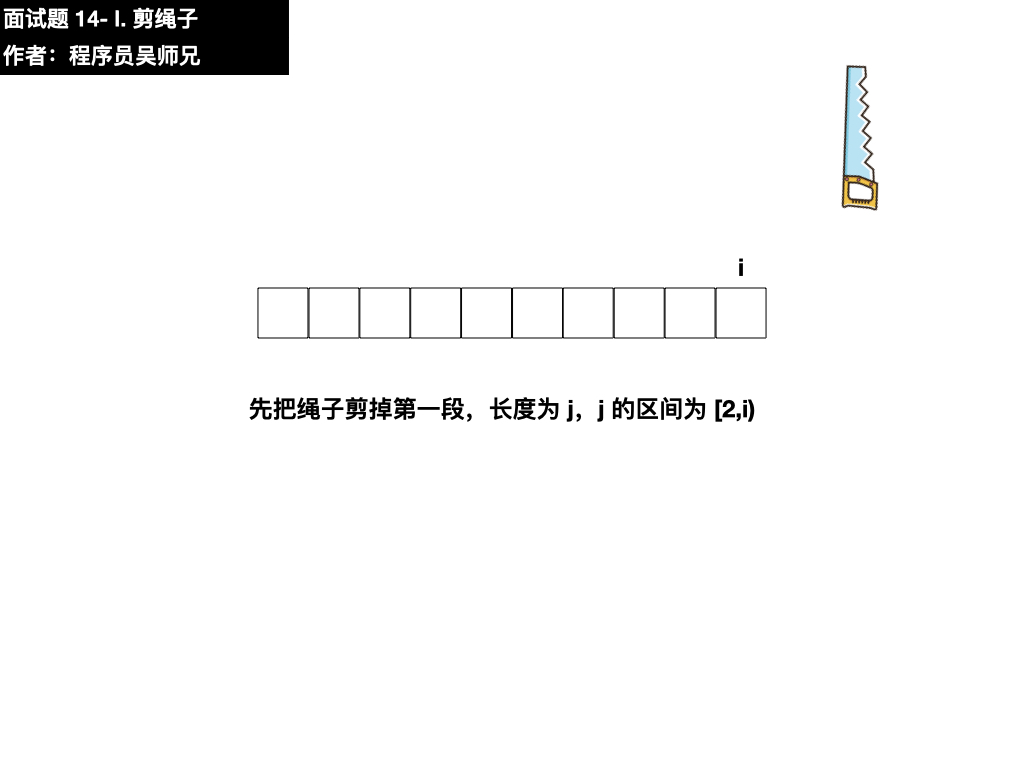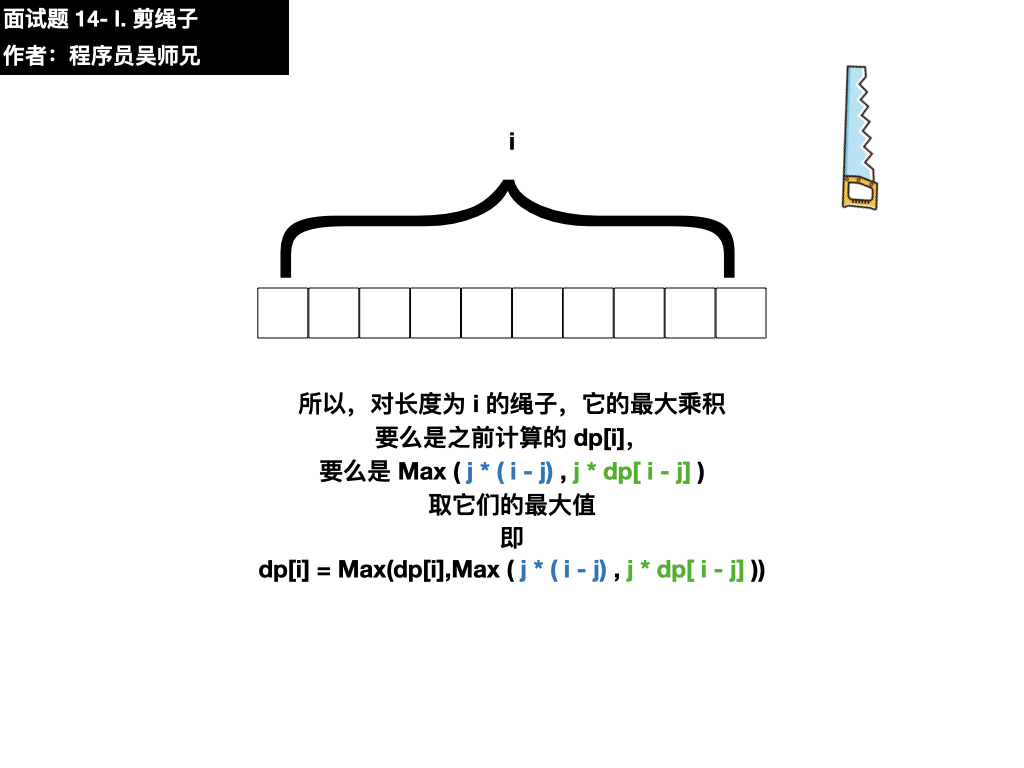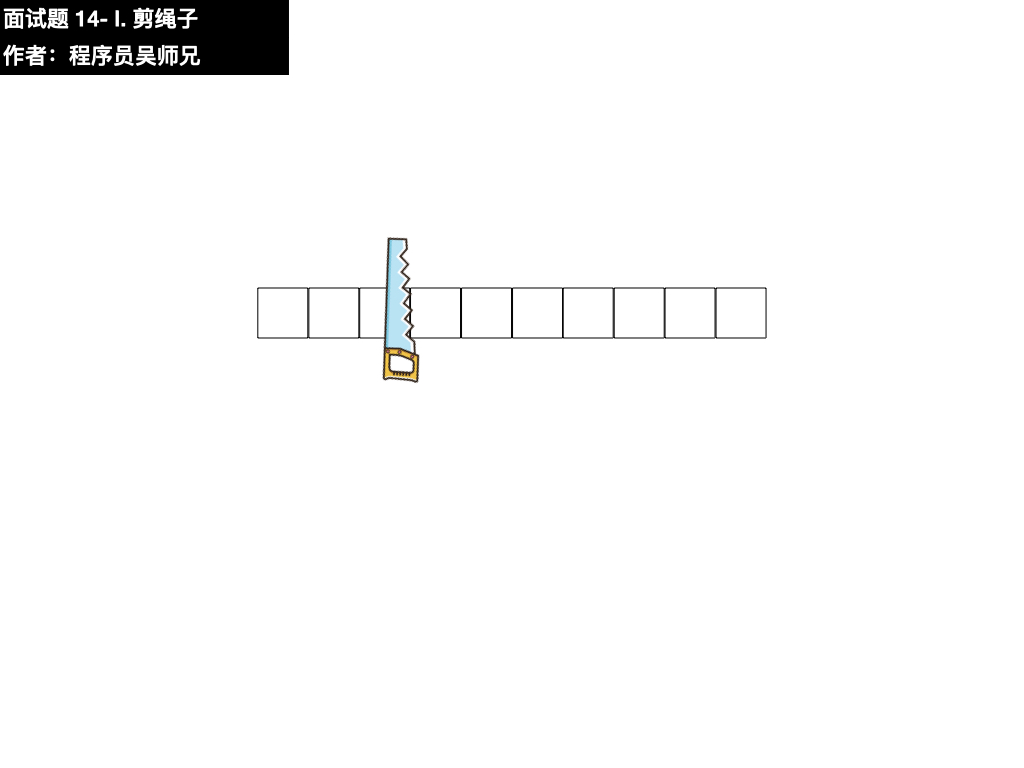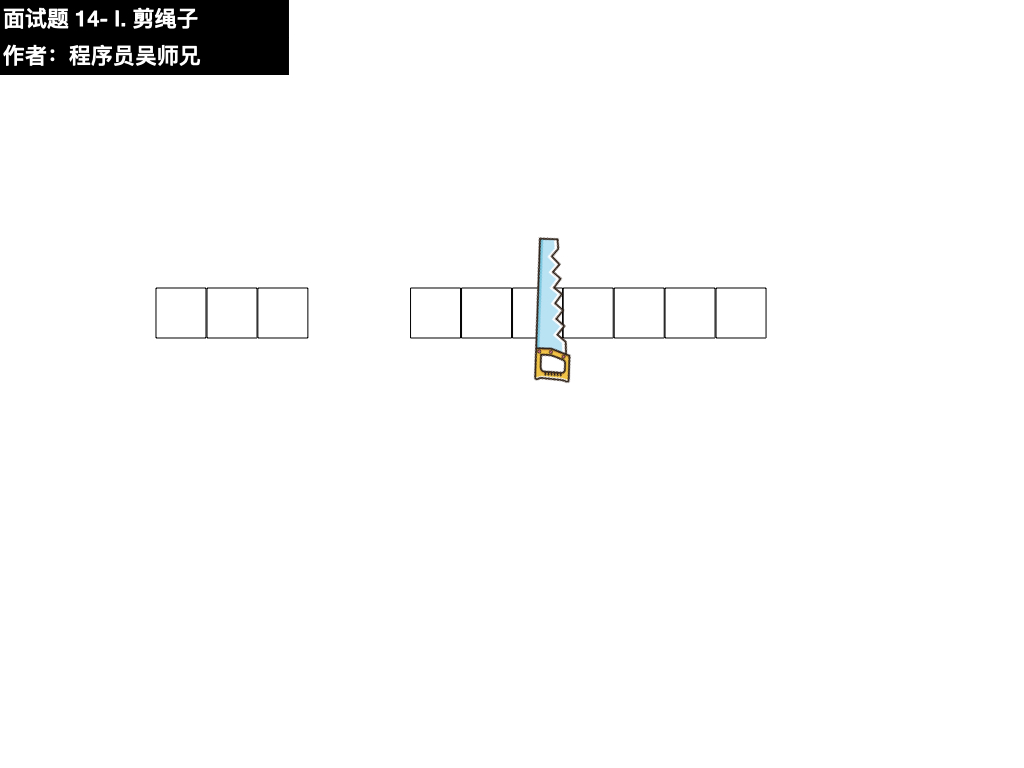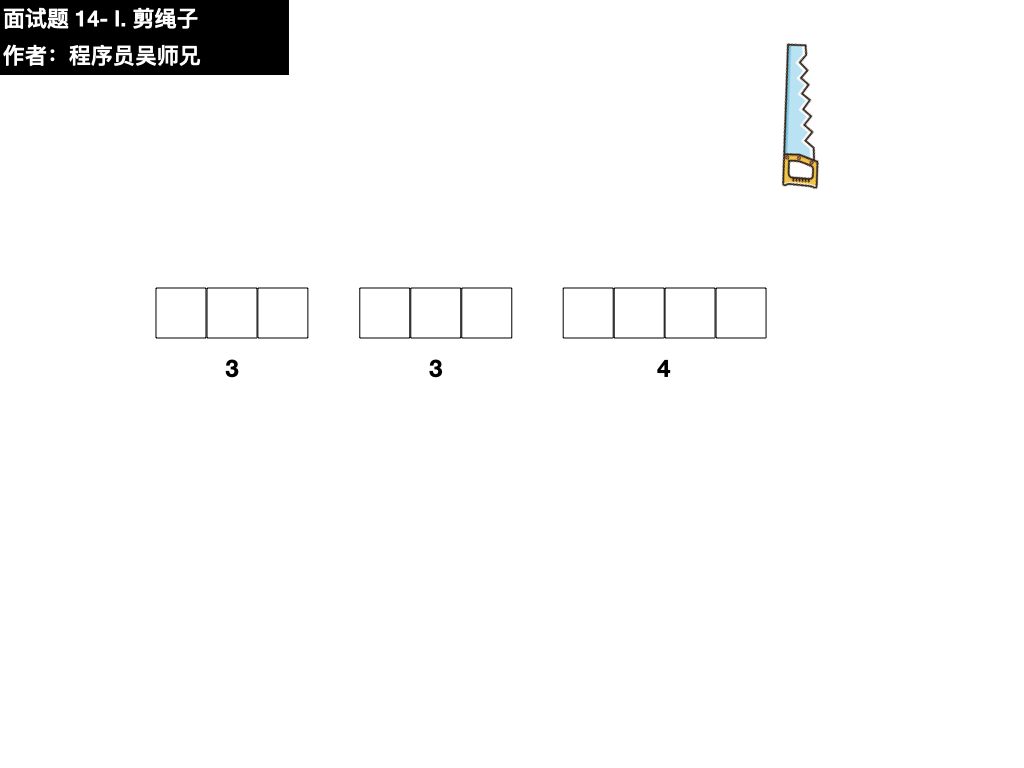### 五、参考代码

// 登录 AlgoMooc 官网获取更多算法图解
// https://www.algomooc.com
class Solution {
public int cuttingRope(int n) {
// 长度为 1 的绳子没法剪了
if ( n <= 1 ) return 1;
// 用一个名为 dp 的数组来记录从 0 到 n 长度的绳子剪掉后的最大乘积
// 默认都是 0
int[] dp = new int[n + 1];
// 由于绳子必须被剪，所以长度为 2 的绳子只有剪成 1 和 1 的两段，乘积结果为 1
dp = 1;
// 长度大于等于 3 的绳子才开始进入我们的讨论范围
// 从长度为 3 的绳子开始考虑，讨论它的最大乘积是多少，并不断的延伸绳子的长度
for(int i = 3; i < n + 1; i++){
// 对于长度为 i 的绳子，它可以分为两个区间 j 和 i - j
// j 的范围由 2 开始，因为剪长度为 1 的绳子无法扩大乘积的值
// j 的范围可以延伸至 i - 1
for(int j = 2; j < i; j++){
// 不剪总长度乘积为  j * （ i - j）
// 剪的话长度乘积为  j * dp[ i - j ]
// 取两者的最大值，即  Max ( j * ( i - j) , j * dp[ i - j] )
// 那么此时 dp[i] 的值取 i 不剪的值（ dp[i]) 和剪的值 Math.max(j * (i - j), j * dp[i - j]) 这两者的最大值
// 比如一开始 i = 3 ， j = 2
// dp = Math.max( 0 ,Math.max ( 2 * 1, 2 * dp)
//       = Math.max( 0 ,Math.max ( 2, 2))
//       = 2
dp[i] = Math.max(dp[i], Math.max(j * (i - j), j * dp[i - j]));
}
}
return dp[n];
}
}


• 动态规划
• 数学

### 八、参考链接

1、https://leetcode-cn.com/problems/jian-sheng-zi-lcof/

2、https://www.algomooc.com/jianzhioffer